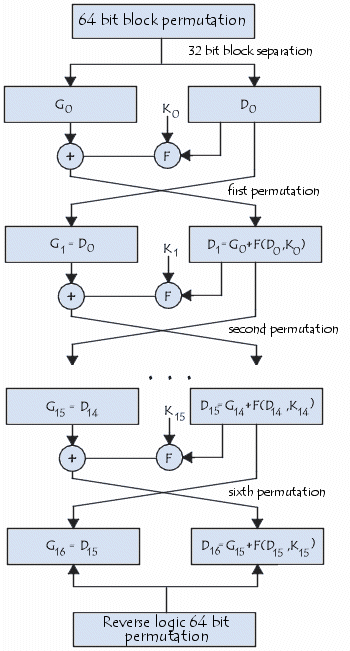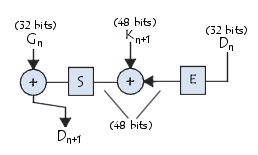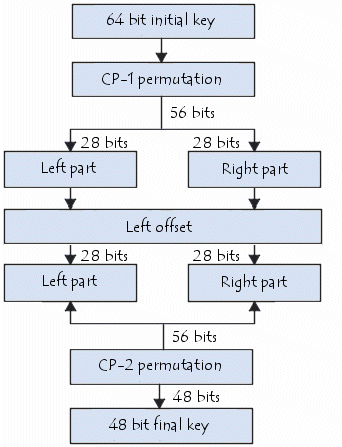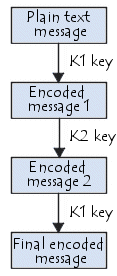# Introduction to Encryption with DES

On May 15, 1973, the NBS (National Bureau of Standards, now called NIST (National Institute of Standards and Technology) published a request in the Federal Register for an encryption algorithm that would meet the following criteria: have a high security level related to a small key used for encryption and decryption; be easily understood; not depend on the algorithm's confidentiality; be adaptable and economical; and be efficient and exportable.

In late 1974, IBM proposed "Lucifer", which was modified on November 23, 1976 to become the DES (Data Encryption Standard). The DES was approved by the NBS in 1978. The DES was standardized by the ANSI (American National Standard Institute) under the name of ANSI X3.92, better known as DEA (Data Encryption Algorithm).## Principle of the DES

The DES is a symmetric encryption system that uses 64-bit blocks, 8 bits (one octet) of which are used for parity checks (to verify the key's integrity). Each of the key's parity bits (1 every 8 bits) is used to check one of the key's octets by odd parity; that is, each of the parity bits is adjusted to have an odd number of '1's in the octet that it belongs to. The key, therefore, has a "useful" length of 56 bits, which means that only 56 bits are actually used in the algorithm.

The algorithm involves carrying out combinations, substitutions, and permutations between the text to be encrypted and the key, while making sure the operations can be performed in both directions (for decryption). The combination of substitutions and permutations is called a product cipher.

The key is ciphered on 64 bits and is made of 16 blocks of 4 bits, generally denoted k1 to k16. Given that only 56 bits are actually used for encrypting, there can be 256 (or 7.2*1016) different keys.

## The DES Algorithm

The main parts of the algorithm are as follows: fractioning of the text</a> into 64-bit (8 octet) blocks; initial permutation of blocks; breakdown of the blocks into two parts: left and right, named L and R; permutation and substitution steps repeated 16 times (called rounds); and re-joining of the left and right parts then inverse initial permutation.## Fractioning of the Text

### Initial permutation

Firstly, each bit of a block is subject to initial permutation, which can be represented by the following initial permutation (IP) table:

IP
 58 50 42 34 26 18 10 2 60 52 44 36 28 20 12 4 62 54 46 38 30 22 14 6 64 56 48 40 32 24 16 8 57 49 41 33 25 17 9 1 59 51 43 35 27 19 11 3 61 53 45 37 29 21 13 5 63 55 47 39 31 23 15 7

This permutation table, when read from left to right, then from top to bottom, shows that the 58th bit of the 64-bit block is in first position, the 50th is in the second position, and so forth.

## Division into 32-Bit Blocks

Once the initial permutation is completed, the 64-bit block is divided into two 32-bit blocks, respectively denoted L and R (for left and right). The initial status of these two blocks is denoted L0 and R0:

L0
 58 50 42 34 26 18 10 2 60 52 44 36 28 20 12 4 62 54 46 38 30 22 14 6 64 56 48 40 32 24 16 8

R0
 57 49 41 33 25 17 9 1 59 51 43 35 27 19 11 3 61 53 45 37 29 21 13 5 63 55 47 39 31 23 15 7

It is noteworthy that L0 contains all bits having an even position in the initial message, whereas R0 contains bits with an odd position

### Rounds

The Ln and Rn blocks are subject to a set of repeated transformations called rounds, shown in this diagram, and the details of which are given below:## Expansion Function

The 32 bits of the R0 block are expanded to 48 bits, thanks to a table called an expansion table (denoted E), in which the 48 bits are mixed together and 16 of them are duplicated:

E
 32 1 2 3 4 5 4 5 6 7 8 9 8 9 10 11 12 13 12 13 14 15 16 17 16 17 18 19 20 21 20 21 22 23 24 25 24 25 26 27 28 29 28 29 30 31 32 1

As such, the last bit of R0 (that is, the 7th bit of the original block) becomes the first, the first becomes the second, and so on.

In addition, bits 1, 4, 5, 8, 9, 12, 13, 16, 17, 20, 21, 24, 25, 28, and 29 of R0 (respectively 57, 33, 25, l, 59, 35, 27, 3, 6l, 37, 29, 5, 63, 39, 31, and 7 of the original block) are duplicated and scattered in the table.

## Exclusive OR with the Key

The resulting 48-bit table is called R0 or E [R0]. The DES algorithm then exclusive ORs the first key K1 with E[R0]. The result of this exclusive OR is a 48-bit table we will call R0 out of convenience (it is not the starting R0!).

### Substitution Function

R0 is, then, divided into 8 6-bit blocks, denoted R0i. Each of these blocks is processed by selection functions (sometimes called substitution boxes or compression functions), generally denoted Si.

The first and last bits of each R0i determine (in binary value) the line of the selection function; the other bits (respectively 2, 3, 4, and 5) determine the column. As the selection of the line is based on two bits, there are 4 possibilities (0, 1, 2, 3). As the selection of the column is based on 4 bits, there are 16 possibilities (0 to 15). Thanks to this information, the selection function "selects" a ciphered value of 4 bits.

Here is the first substitution function, represented by a 4-by-16 table:

S1
0123456789101112131415
01441312151183106125907
10157414213110612119538
24114813621115129731050
31512824917511314100613

Let R01 equal 101110. The first and last bits give 10, that is, 2 in binary value. The bits 2, 3, 4, and 5 give 0111, or 7 in binary value. The result of the selection function is therefore the value located on line no. 2, in column no. 7. It is the value 11, or 111 binary.

Each of the 8 6-bit blocks is passed through the corresponding selection function, which gives an output of 8 values with 4 bits each. Here are the other selection functions:

S2
0123456789101112131415
01518146113497213120510
13134715281412011069115
20147111041315812693215
31381013154211671205149

S3
0123456789101112131415
01009146315511312711428
11370934610285141211151
21364981530111212510147
31101306987415143115212

S4
0123456789101112131415
07131430691012851112415
11381156150347212110149
21069012117131513145284
331506101138 94511127214

S5
0123456789101112131415
02124171011685315130149
11411212471315015103986
24211110137815912563014
31181271142136150910453

S6
0123456789101112131415
01211015926801334147511
11015427129561131401138
29141552812370410113116
34321295151011141760813

S7
0123456789101112131415
04112141508133129751061
11301174911014351221586
21411131237141015680592
36111381410795015142312

S8
0123456789101112131415
01328461511110931450127
11151381037412561101492
17114191214206101315358
12114741081315129035611

Each 6-bit block is, therefore, substituted in a 4-bit block. These bits are combined to form a 32-bit block.

### Permutation

The obtained 32-bit block is, then, subject to a permutation P. Here is the table:

P
 16 7 20 21 29 12 28 17 1 15 23 26 5 18 31 10 2 8 24 14 32 27 3 9 19 13 30 6 22 11 4 25

## Exclusive OR

All of these results output from P are subject to an Exclusive OR, with the starting L0 (as shown on the first diagram) to give R1, whereas the initial R0 gives L1.

### Iteration

All of the previous steps (rounds) are repeated 16 times.

### Inverse Initial Permutation

At the end of the iterations, the two blocks L16 and R16 are re-joined, then subject to inverse initial permutation:

IP-1
 40 8 48 16 56 24 64 32 39 7 47 15 55 23 63 31 38 6 46 14 54 22 62 30 37 5 45 13 53 21 61 29 36 4 44 12 52 20 60 28 35 3 43 11 51 19 59 27 34 2 42 10 50 18 58 26 33 1 41 9 49 17 57 25

The output result is a 64-bit ciphertext.

## Generation of Keys

Given that the DES algorithm presented above is public, security is based on the complexity of encryption keys.

The algorithm below shows how to obtain, from a 64-bit key (made of any 64 alphanumeric characters), 8 different 48-bit keys each used in the DES algorithm:Firstly, the key's parity bits are eliminated so as to obtain a key with a useful length of 56 bits.

The first step is a permutation denoted PC-1 whose table is presented below:

PC-1
 57 49 41 33 25 17 9 1 58 50 42 34 26 18 10 2 59 51 43 35 27 19 11 3 60 52 44 36 63 55 47 39 31 23 15 7 62 54 46 38 30 22 14 6 61 53 45 37 29 21 13 5 28 20 12 4

This table may be written in the form of two tables Li and Ri (for left and right) each made of 28 bits:

Li
 57 49 41 33 25 17 9 1 58 50 42 34 26 18 10 2 59 51 43 35 27 19 11 3 60 52 44 36

Ri
 63 55 47 39 31 23 15 7 62 54 46 38 30 22 14 6 61 53 45 37 29 21 13 5 28 20 12 4

The result of this first permutation is denoted L0 and R0.

These two blocks are, then, rotated to the left, such that the bits in second position take the first position, those in third position take the second, etc.

The bits in first position move to last position.

The two 28-bit blocks are, then, grouped into one 56-bit block. This passes through a permutation, denoted PC-2, giving a 48-bit block as output, representing the key Ki.

PC-2
 14 17 11 24 1 5 3 28 15 6 21 10 23 19 12 4 26 8 16 7 27 20 13 2 41 52 31 37 47 55 30 40 51 45 33 48 44 49 39 56 34 53 46 42 50 36 29 32

Repeating the algorithm makes it possible to give the 16 keys K1 to K16 used in the DES algorithm.

LS
 1 2 4 6 8 10 12 14 15 17 19 21 23 25 27 28

## TDES, an Alternative to the DES

In 1990, Eli Biham and Adi Shamir developed differential cryptanalysis, which searches for plaintext pairs and ciphertext pairs. This method works with up to 15 rounds, while 16 rounds are present in the algorithm presented above.

Moreover, while a 56-bit key gives an enormous amount of possibilities, many processors can compute more than 106 keys per second; as a result, when they are used at the same time on a very large number of machines, it is possible for a large body (a State for example) to find the right key.

A short-term solution involves catenating three DES encryptions using two 56-bit keys (which equals one 112-bit key). This process is called Triple DES, denoted TDES (sometimes 3DES or 3-DES).TDES is much more secure than DES, but it has the major disadvantage of also requiring more resources for encryption and decryption.

Several types of triple DES encryption are generally recognized: DES-EEE3: 3 DES encryptions with 3 different keys; DES-EDE3: a different key for each of the 3 DES operations (encryption, decryption, encryption); and DES-EEE2 and DES-EDE2, a different key for the second operation (decryption).

In 1997, NIST launched a new call for projects to develop the AES (Advanced Encryption Standard), an encryption algorithm intended to replace the DES.

The DES encryption system was updated every five years. In 2000, during the most recent revision, after an evaluation process that lasted for three years, the algorithm that was jointly designed by two Belgian candidates Sirs Vincent Rijmen and Joan Daemen was chosen as the new standard by NIST. This new algorithm, named RIJNDAEL by its inventors, will replace the DES from now on.

Image: © Signs and Symbols - Shutterstock.com